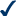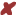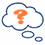• # Order of Operations - PEMDAS

## Operations

"Operations" means things like add, subtract, multiply, divide, squaring, etc. If it isn't a number it is probably an operation.

But, when you see something like ...

7 + (6 × 52 + 3)

... what part should you calculate first?

Start at the left and go to the right?
Or go from right to left?

Warning: Calculate them in the wrong order, and you will get a wrong answer !

So, long ago people agreed to follow rules when doing calculations, and they are:

## Order of Operations

Do things in Parentheses First. Example:6 × (5 + 3) = 6 × 8 = 486 × (5 + 3) = 30 + 3 = 33 (wrong)

Exponents (Powers, Roots) before Multiply, Divide, Add or Subtract. Example:5 × 22 = 5 × 4 = 205 × 22 = 102 = 100 (wrong)

Multiply or Divide before you Add or Subtract. Example:2 + 5 × 3 = 2 + 15 = 172 + 5 × 3 = 7 × 3 = 21 (wrong)

Otherwise just go left to right. Example:30 ÷ 5 × 3 = 6 × 3 = 1830 ÷ 5 × 3 = 30 ÷ 15 = 2 (wrong)

## How Do I Remember It All ... ? PEMDAS !

 P Parentheses first E Exponents (ie Powers and Square Roots, etc.) MD Multiplication and Division (left-to-right) AS Addition and Subtraction (left-to-right)

Divide and Multiply rank equally (and go left to right).

Add and Subtract rank equally (and go left to right)After you have done "P" and "E", just go from left to right doing any "M" or "D" as you find them. Then go from left to right doing any "A" or "S" as you find them.You can remember by saying "Please Excuse My Dear Aunt Sally".   For more examples visit http://www.mathsisfun.com/operation-order-pemdas.html